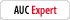# Calculating totals returning error if any cell is empty.

kazoom
Registered: Sep 24 2008
Posts: 3

I am using a Custom Script to total a column of figures.

-------------------------------------------
var f0=this.getField("txAmount0").value;
var f1=this.getField("txAmount1").value;
var f2=this.getField("txAmount2").value;
var f3=this.getField("txAmount3").value;

event.value=f0 + f1 + f2 + f3;

if (event.value==0)
event.value="";

----------------------------------------------

This works correctly unless there is an empty field between two fields with entries, then it stops adding to itself (to increase the total) and starts to tack on the new entries to the end of the old!

For example: if I put the figure 10 into the first three fields. the total is correctly displayed as 30.

However, if I only put the number 10 into fields 1 and 3 (leaving field 2 empty) the total displays like this : 1,010.00 and continues to add on each entry.

If I go back and put a number into the blank field, even a zero, the total recalculates and displays correctly.

So there is obviously something wrong with my calculation script. i have tried pretty well every variation I can think of to get it to work, but no luck.

I'm sure there is a simple solution, but it's eluding me!

I need to have to 0.00 default display hidden because the form is sometimes printed to be filled out manually, so the 0.00 spoilse the total field.

I'd be grateful for any help... even if I have to say DOH! when the answer is revealed :-)

Version is Acrobat 9 Pro Extended 9.4.6

Thank you

My Product Information:
Acrobat Pro Extended 9.4.3, Windows
try67Registered: Oct 30 2008
Posts: 2398
When the field is empty it returns an empty string (""). The "+" operator is used to both add up numbers and to concatenate strings. So when the script sees that one of the values is a string, it defaults to treating them all as such, and therefore the values get concatenated.
The solution is simple: force the script to treat the blank values as a number (0), by placing a Number() constructor around them, or simply a "+" before them (another use for it!), like so:

var f0 = +this.getField("txAmount0").value;
var f1 = +this.getField("txAmount1").value;
var f2 = +this.getField("txAmount2").value;
var f3 = +this.getField("txAmount3").value;

- AcrobatUsers Community Expert - Contact me personally at try6767 [at] gmail [dot] com
Check out my custom-made scripts website: http://try67.blogspot.com

kazoom
Registered: Sep 24 2008
Posts: 3
Thank you very much try67, your solution worked perfectly :-)

Now then, one extra little question:

The extended script I use on the form has 21 fields, so the calculating script looks like this:

-----------------------------------------------

var f0 = +this.getField("txAmount0").value;
var f1 = +this.getField("txAmount1").value;
var f2 = +this.getField("txAmount2").value;
var f3 = +this.getField("txAmount3").value;
var f4 = +this.getField("txAmount4").value;
var f5 = +this.getField("txAmount5").value;
var f6 = +this.getField("txAmount6").value;
var f7 = +this.getField("txAmount7").value;
var f8 = +this.getField("txAmount8").value;
var f9 = +this.getField("txAmount9").value;
var f10 = +this.getField("txAmount10").value;
var f11 = +this.getField("txAmount11").value;
var f12 = +this.getField("txAmount12").value;
var f13 = +this.getField("txAmount13").value;
var f14 = +this.getField("txAmount14").value;
var f15 = +this.getField("txAmount15").value;
var f16 = +this.getField("txAmount16").value;
var f17 = +this.getField("txAmount17").value;
var f18 = +this.getField("txAmount18").value;
var f19 = +this.getField("txAmount19").value;
var f20 = +this.getField("txAmount20").value;

event.value=f0 + f1 + f2 + f3 + f4 + f5 + f6 + f7 + f8 + f9 + f10 + f11 + f12 + f13 + f14 + f15 + f16 + f17 + f18 + f19 + f20;

if(event.value==0)
event.value="";
---------------------------------------------------

Is this the best format of the script or is there a more efficient (shorthand) format that can be used?

No problem as it is, just wondering :-)
kazoom
Registered: Sep 24 2008
Posts: 3
Something to remember when dealing with Acrobat calculations I have just discovered.

After replacing the 'Value is the...' script with the Custom Script above, my 'Total Return' script began to return 10 fewer than it should!

Then I checked the Calculation Order (Forms > Edit Fields > Set Field Calculation Order...) and discovered the TotalSpent and the Return fields had swapped round.Reordering the fields correctly for the calculation, fixed it.

Trap for young players, I was lucky I had read something about that issue earlier in the day or I would have been stumped...again!
gkaiserilRegistered: Feb 23 2006
Posts: 4307
You can reduce the size of your addition script by using a control loop and computing the field name. You can also use the Number constrictor to force a null field to the number zero. Using a function will also reduce the run time of the script.

(function() {
var Sum = 0; // variable for sum
// control loop to sum fields
for(i = 0; i < 20; i++) {
Sum += Number(this.getField("txtAmount" + i).value); // add i field
} // end for loop
if(Sum == 0) Sum = ""; // null value if Sum is zero
return Sum; // return result
}) (); // run function

George Kaiser

George_JohnsonRegistered: Jul 6 2008
Posts: 1875
Using the unary + operator as try67 showed is generally the fastest way to convert a string to a number, while using the Number constructor is equivalent in outcome but is generally the slowest method. Unary + is also results in smaller and more readable (IMO) code, but some find the Number constructor is more clear and worth whatever speed penalty there may be. For more info, see: http://jibbering.com/faq/notes/type-conversion/#tcNumber
try67Registered: Oct 30 2008
Posts: 2398
Consider this, as well: If all you're doing is adding up these fields, you might as well use the built-in method for doing that ("Value is the (Sum) of fields..."), and then enter this as the custom validation script to avoid displaying the zero value:

if (event.value==0) event.value="";

- AcrobatUsers Community Expert - Contact me personally at try6767 [at] gmail [dot] com
Check out my custom-made scripts website: http://try67.blogspot.com